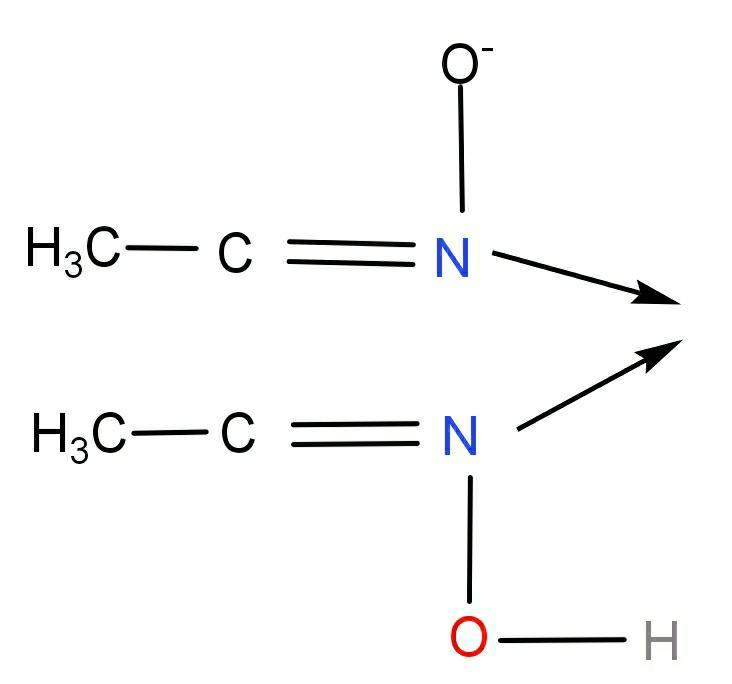What is the number of donor atoms in dimethylglyoximato ligand ?(A) 1(B) 2(C) 3(D) 4Verified
146.4k+ views
Hint: There are more than one donor atoms in dimethylglyoximato. Nitrogen has a lone pair of electrons while oxygen in dimethylglyoximato is involved in hydrogen bonding. It is also called bidentate ligand.

To find the number of donor atoms in dimethylglyoximato, first we can give a close observation on the structure of dimethylglyoximato.In this compound, Both the Nitrogen atoms are donors. O is involved in hydrogen bonding and because of that reason Oxygen atoms cannot be a donor.

So, it is clear that there are two donor atoms (N and N) in dimethylglyoximato.

Therefore the number of donor atoms in dimethylglyoximato is 2.

Option (B) 2 is the correct answer.

Dimethylglyoxime (DMG) is used as an analytical reagent used to precipitate Ni from its aqueous solution. Here Nickel complexes with dmg form octahedral, square planar and tetrahedral complexes in +2 oxidation state. The hybridization of Ni in the complex , $\left[ Ni{ \left( DMG \right) }_{ 2 } \right]$ is ${ dsp }^{ 2 }$.
${ Ni }^{ 2+ }\ +\ { 2DMG\ \left( dimethylglyoxime \right) \ \rightarrow \ \left[ Ni{ \left( DMG \right) }_{ 2 } \right] }\downarrow \ \left( bright\ red \right)$
Chelation and intramolecular hydrogen bonding are the two main factors which provide stability to this compound. Oxidation state of Ni in $\left[ Ni{ \left( DMG \right) }_{ 2 } \right]$ is +2 and has a square planar geometry because of chelation the pairing of electrons takes place. As all electrons are paired so this complex is diamagnetic and nickel with coordination number four.